# KSEEB Solutions for Class 5 Maths Chapter 8 Length

Students can Download Maths Chapter 8 Length Questions and Answers, Summary, Notes Pdf, KSEEB Solutions for Class 5 Maths helps you to revise the complete Karnataka State Board Syllabus and score more marks in your examinations.

## Karnataka State Syllabus Class 5 Maths Chapter 8 Length

### KSEEB Class 5 Maths Length Ex 8.1

Question 1.
How many millimeters make one centimeter?
10 millimeters

Question 2.
How many centimeters make one meter?
1m = 100cmQuestion 3.
How many meters make one kilometer?
1 kilometre = 1000 metres

Question 4.
How many meters make half a kilometer?
500 meters

Question 5.
How many centimetres make $$\frac{3}{4}$$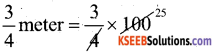= 75 centimetersII. Solve the following:

Question 1.
Convert 573cm into meters.
1 meter = 100 Centimeter
573cm = 573/100 = 5.73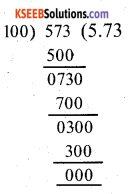Question 2.
Convert 1,378m into kilometers.
1 kilometer = 1000 meter
1378 meter = 1378/1000
= 1.378 kilometer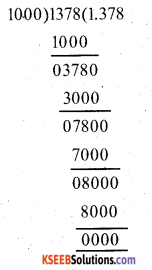Question 3.
Convert 1,515 cm into millimeters.
1cm = 10 millimeter
1515cm = 1515 × 10
= 15150 millimeterQuestion 4.
Radha’s school is at a distance of 2,450m from her house. Express this distance in km.
1 kilometer = 1000 meter
Radha’s school is at a distance of 2,450mt
The distance in km = 2400/1000
= 2.450 kilometers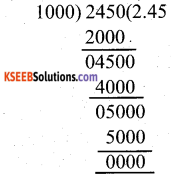Question 5.
The length of a ground is 15 meters. Express the length in centimeters.
1 meter = 100 centimeter
The length of a ground = 15 meters
The length in centimeter = 100 × 15 = 1500### KSEEB Class 5 Maths Length Ex 8.2

Question 1.
The length of a wire is 3m. What is the total length of 5 such wire?
The length of a wire is 3 meter The total length of 5 such wire = 5 × 3
= 15 metersQuestion 2.
Out of a roll of cloth measuring 50m, 17m of cloth is sold. Find the length of the remaining cloth.
A roll of cloth measure 50 meters 17 meter of cloth is sold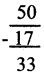The remaining length of cloth = 33 meterQuestion 3.
24m of colored ribbon is distributed among 8 students equally. What is the length of the ribbon that each one gets?
The number of students = 8
The colored ribbons = 24 meter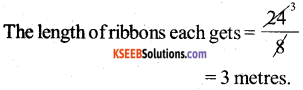Question 4.
How many centimeters are there in 9 meters?
1 metre = 100 centimetre
9 metre 100 × 9
= 900 centimeterQuestion 5.
The length of a wooden plank is 19m. Out of this 5 pieces of 3tn each is cut off. Find the length of the remaining piece.
The length of a wooden plank = 19 metre
5 pieces of 3 metre each cut off = 5 × 3 = 15

The length of the remaining piece
= 19 – 15
= 4 meter1. 22m 71cm and 14m 30cm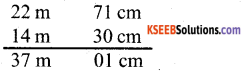2. 4km 230m and 22km 280m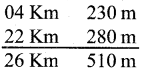III. Subtract the following:

1. 68m 35cm from 75m 48cm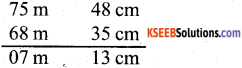2. 12km 425m from 17km 650m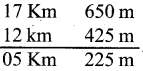IV. Solve the following:

Question 1.
The length of a line segment is 12cm. Into how many line segments of 3cm each, can it be divided?
The length of a line segment = 12cm
Each line segment = 3cm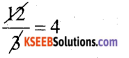The length of each line segment = 4cm2. Ravi bought 3m 60cm length of pant cloth. He gave lm 20 cm of cloth to his younger brother. What is the length of the remaining cloth?
Ravi bought of pant cloth – 3m, 60cm Ravi gave cloth to his younger brother . = 1m, 20cm
The length of the remaining cloth = ( 3m- lm) (60cm- 20cm)
= 2m, 40cmQuestion 3.
The length of cloth purchased by John is as follows: 2m 20cm for shirt, 1m 20 cm for pant and 4m 80cm for coat. What is the total length of cloth purchased by John?
The length of cloth purchased by John
2 m 20cm for shirt
1 m 20cm for pant
4 m 80cm for coat
8 m 20 cm
1 m = 100 centimetre
Total length of cloth purchased by john = 8m, 20cmQuestion 4.
2m 80cm of cloth is required to stitch a frock. What is the length of cloth required to stitch 12 such frocks?
To stitch a frock = 2m, 80cm
The length of cloth repaired to stitch 12
such frocks = 12 x 2 = 24
= $$\frac{12 \times 80}{960 \mathrm{cm}}$$
1 m = 100cm
960 cm = $$\frac{960}{100}$$ = 9.6m
24 metre + 9 metre + 60 cemtimetre 33 metres, 60 centimetresQuestion 5.
The length of cloth required to stitch 6 pairs of Kurta and Pyjama is 33m. What is the length of cloth required to stitch 1 pair of Kurta & Pyjama?
Length of cloth required to stitch 6 pairs
of kurtha & pyjama = 33m
The length of cloth required to stitch are

pair of kurtha & pyjama $$\frac{33}{6}=5.5$$Question 6.
The total length of 8 bundles of wire is 204m. Find the length of each bundle of wire.
The total length of 8 bundles of wire = 204m
The length of each bundle of wire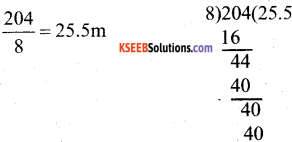Question 7.
The hight of a table is 1 m 25cm and the height of a stool is 50cm less than the height of the table. Find the height of the stool.
The height of a table = 1 m + 25cm
The height of a stoll = 50 cm less than the height of table
= (1m + 25cm) – 50 cm = 75cmQuestion 8.
A gram panchayat constructed a road to connect the village and the main road. On the first day 3 km 460 m of road was constructed and 4 km 540m of road was constructed on the next day. What is the total length of the road constructed?